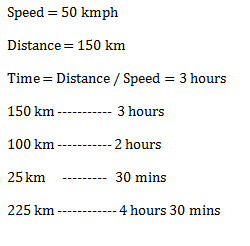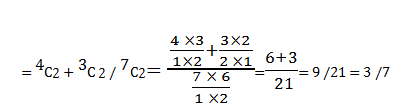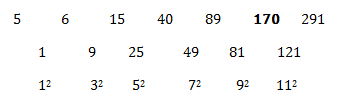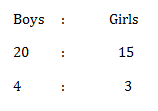# SSC CGL 2018 Practice Test Papers | Quantitative Aptitude (Day-59)

Dear Aspirants, Here we have given the Important SSC CGL Exam 2018 Practice Test Papers. Candidates those who are preparing for SSC CGL 2018 can practice these questions to get more confidence to Crack SSC CGL 2018 Examination.

[WpProQuiz 3730]

Click “Start Quiz” to attend these Questions and view Explanation

1) What is the Area of triangle whose base is 12 cm and height is 16 cm?

a) 74

b) 64

c) 96

d) 78

2) Find the value of x
25% of 720 + 75% of 560 = 4x

a) 220

b) 200

c) 100

d) 150

3) A and B can do a piece of work in 10 days and B and C can do the same job in 15 days. In how many days A and C can finish the work?

a) 12 days

b) 16 days

c) 14 days

d) Cannot be determined

4) A Train runs with the speed of 50 kmph and reached 150km. In how long it takes to cover the distance of 225 km?

a) 4 hours

b) 4.5 hours

c) 5 hours

d) 5. 5 hours

5) A bag contains 4 blue balls and 3 red balls. What is the probability of same colour balls picked up, if two balls are picked up randomly?

a) 6/7

b) 11/42

c) 3/ 7

d) 4 / 7

6) The Product of two co-prime numbers is 101. Their L.C.M should be

a) 1

b) 101

c) 0

d) Cannot be determined

7) Preeti sold her laptop with the loss of 10%. If she sells for Rs. 1500 more she got 10 % gain on this laptop. Find the cost price of the laptop.

a) 10000

b) 12500

c) 7500

d) 2450

8) Find the missing term of the given series

5, 6, 15, 40, 89, ?, 291

a) 180

b) 170

c) 160

d) 150

9) In a class there are 15 girls and 20 boys. What is the ratio of boys to that of girls?

a) 3 : 4

b) 4: 5

c) 4: 3

d) 5: 6

10) ¾ of 5/6of 11/ 7 of a number is 495. What is that number?

a) 550

b) 110

c) 504

d) 546

Area of triangle = ½ Base × height

= ½ × 12 × 16 = 12 × 8 = 96 cm2

= 4x => = 4x

= 18 + 42 = 4x

4x = 600

X = 150

A + B = 1/ 10 days

B + C = 1/15 days

We have only 2 equations but 3 variables are there. So we cannot conclude this sum.Probability of same colour balls picked upH.C.F of co-prime numbers is 1.

L.C.M = 101/1 =101

Let C. P = 100

1. P = 90

If he gains 10%, then S.P = 110

110 – 90 = 20

1500 * 100/ 20 = 7500